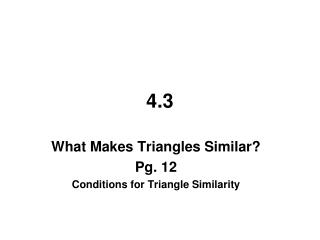# 4.3 - PowerPoint PPT PresentationDownload Presentation4.3

4.3
Download Presentation## 4.3

- - - - - - - - - - - - - - - - - - - - - - - - - - - E N D - - - - - - - - - - - - - - - - - - - - - - - - - - -
##### Presentation Transcript

1. 4.3 What Makes Triangles Similar? Pg. 12 Conditions for Triangle Similarity

2. 4.3–What Makes Triangles Similar? Conditions for Triangle Similarity Now that you know what similar shapes have in common, you are ready to turn to a related question: How much information do I need to know that two triangles are similar? As you work through today's lesson, remember that similar polygons have corresponding angles that are equal and corresponding sides that are proportional.

3. 4.21 – ARE THEY SIMILAR? Erica thinks the triangles below might be similar. However, she knows not to trust the way figures look in a diagram, so she asks for your help.

4. a. If two shapes are similar, what must be true about their angles? Corresponding angles must be congruent b. What must be true about their sides? Corresponding sides must be proportional

5. b. Measure the angles and sides of Erica's Triangles and help her decide if the triangles are similar or not. 40° 5.6cm 2cm 20° 120° 40° 4.3cm 11.2cm 4cm 120° 20° 8.6cm Similar

6. 4.22 – HOW MUCH IS ENOUGH? Jessica is tired of measuring all the angles and sides to determine if two triangles are similar. "There must be an easier way," she thinks.

7. http://hotmath.com/util/hm_flash_movie_full.html?movie=/hotmath_help/gizmos/triangleSimilarity.swfhttp://hotmath.com/util/hm_flash_movie_full.html?movie=/hotmath_help/gizmos/triangleSimilarity.swf http://hotmath.com/util/hm_flash_movie_full.html?movie=/hotmath_help/gizmos/triangleSimilarity.swf

8. Side-Side-Side Similarity: If all 3 corresponding sides are proportional, then the triangles are similar E B A C D F

9. Angle-Angle Similarity: If 2 corresponding angles are congruent, then the triangles are similar E B A C D F

10. 4.23 – WHAT'S YOUR ANGLE? Determine whether the triangles are similar. If they are, explain why and write a similarity statement.

11. 77° 55° AA~

12. 47° 31°

13. AA~

14. AA~

15. 4.24 – ARE THEY SIMILAR? Based on your conclusions, decide if each pair of triangles below are similar. If they are make a similarity statement. Then determine if you are using Angle-Angle similarity or Side-Side-Side similarity.

16. SSS~

17. 52° 96° AA~

18. d. AA~ Yes,

19. 4.25 – FLOWCHARTS Examine the triangles at right. a. Are these triangles similar? Why? Yes, AA~

20. b. Julio decided to use a diagram (called a flowchart) to explain his reasoning. Compare your explanation to Julio's flowchart. Did Julio use the same reasoning you used? Yes

21. c. What appears to go in the bubbles of a flowchart? What goes outside the bubbles? Inside: Statements Outside: Reasons

22. 4.26 – WRITING FLOWCHARTS Besides showing your reasoning, a flowchart can be used to organize your work as you determine whether or not triangles are similar. a. Are these triangles similar? Why? Yes, SSS~

23. b. What facts must you know to use the triangle similarity conjecture you chose? Julio started to list the facts in a flowchart at right. Complete the third oval.

24. c. Once you have the needed facts in place, you can conclude that you have similar triangles. Add to your flowchart by making an oval and filling in your conclusion.

25. d. Finally, draw arrows to show the flow of the facts that lead to your conclusion and record the similarity conjecture you used, following Julio's example.

26. 4.27 – START FROM SCRATCH a. What is the best conjecture to test for these triangles? SSS~ or AA~? AA~, Only know angles

27. b. Are these triangles similar? Justify your conclusion using a flow chart.

28. given ΔJKL ~ ΔYZX AA~

29. 4.27 – HOW MANY OVALS? a. What is the best conjecture to test for these triangles? SSS~ or AA~? SSS~, Only know sides

30. b. Are these triangles similar? Justify your conclusion using a flow chart.

31. 12 8 3 2 9 6 3 2 15. 10 3 2 = = = given given given ΔABC ~ ΔDEF SSS~